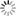# Episode List

OR

### 1960

1 Jan. 1960
Life in the BalanceBe the first one to add a plot.

8 Jan. 1960
KarateBe the first one to add a plot.

15 Jan. 1960
Blue FireBe the first one to add a plot.

22 Jan. 1960
My Brother's KeeperBe the first one to add a plot.

29 Jan. 1960
House Call
7.7 (7)Be the first one to add a plot.

5 Feb. 1960
The TrapA detective goes undercover to capture a cop killer.

12 Feb. 1960
Conspiracy of SilenceBe the first one to add a plot.

19 Feb. 1960
Twelve Hours to LiveBe the first one to add a plot.

26 Feb. 1960
Anatomy of FearBe the first one to add a plot.

4 Mar. 1960
Armed and DangerousBe the first one to add a plot.

11 Mar. 1960
The Bad Eye of Rose RosettiBe the first one to add a plot.

18 Mar. 1960
Time and TideBe the first one to add a plot.

25 Mar. 1960
Little Girl LostBe the first one to add a plot.

1 Apr. 1960
The Chameleon TruckBe the first one to add a plot.

8 Apr. 1960
The Old GangBe the first one to add a plot.

15 Apr. 1960
The BodyguardsBe the first one to add a plot.

22 Apr. 1960
The ProwlerBe the first one to add a plot.

29 Apr. 1960
Floating Face Down
8.0Be the first one to add a plot.

6 May 1960
The Long JumpBe the first one to add a plot.

13 May 1960
Trial by FireBe the first one to add a plot.

20 May 1960
The Retirement of Maria MuirEva Gabor portrays an award winning actress who is a possible potential murder victim? Or could she be the murderer herself?

25 May 1960
Song of SongsBe the first one to add a plot.

16 Sep. 1960
The New ManBe the first one to add a plot.

23 Sep. 1960
LongshotBe the first one to add a plot.

30 Sep. 1960
The Sports JobBe the first one to add a plot.

7 Oct. 1960
AlibisBe the first one to add a plot.

14 Oct. 1960
ShuttleBe the first one to add a plot.

4 Nov. 1960
Cop on TrialBe the first one to add a plot.

11 Nov. 1960
The Little WitnessBe the first one to add a plot.

18 Nov. 1960
You Only Die OnceBe the first one to add a plot.

25 Nov. 1960Be the first one to add a plot.

2 Dec. 1960
The Other SideBe the first one to add a plot.

9 Dec. 1960
The ScalpelBe the first one to add a plot.

16 Dec. 1960
Big PoisonBe the first one to add a plot.

23 Dec. 1960
The InformerBe the first one to add a plot.

30 Dec. 1960
Razor's EdgeThe sumptuous interiors of the Norman Palace are your entry point to Palermo and its rich multicultural history. Grasp Norman Sicily's historical role as the richest and best-governed state in the West. Explore the complex architecture of the city's churches, cathedral, and Palazzo Abatellis, and the cosmopolitan past of the seaport.

« 1959 |  1960  1961  »